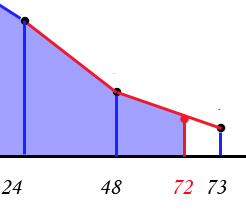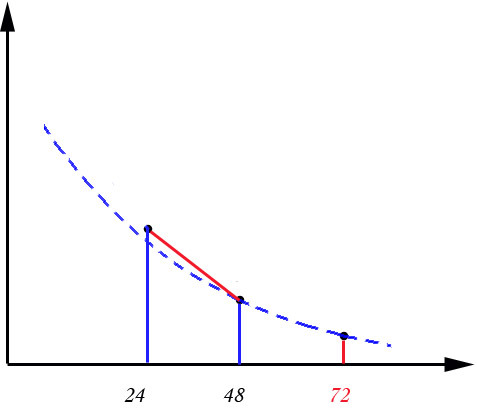## Partial AUC calculation [NCA / SHAM]

Dear all!
Sorry for reviving the battered topic but for validation purposes I need to understand the methods of calculating partial areas in some nasty cases. I have a premonition that it would be hard to understand my question so I’ll try to use more illustrations…

Suppose we have to calculate AUC0-72. The biggest problem is to decide: to extrapolate or nor to extrapolate. Let us follow the first scene. Suppose that the protocol states to use a simple linear trapezoid method to calculate AUC. How to calculate AUC0-72 in the following situations?
1. There were time deviations in the first point (that is instead of t0=0, we have, for example, t0=1 hour)
2. There were time deviations in the last sample point (that is instead of tlast=72 h we have tlast=71 h or tlast=73 h). Additionally what if we have concentrations below LLOQ in the several last points (agree it is strange for drugs with long half-life but who knows..)?
3. There were abscent samples (NA) at the last time point 72 h
As Phoenix WinNonLin® presents itself as a golden standard of NCA, I tried to understand what methods does it use in the uppermentioned cases.

To compare different methods I used the following concentration-time table:time      Conc.  ---------------------------------- 0    0 (NA) 1   3 2   17 3   55 4   87 5   190 6   250 7   315 8   330 10   230 12   185 16   115 18   100 24   125 48   100 72(71,73)    77 (NA)  ----------------------------------1. Let us start with the first case: as it is intuitevily simple we just use a linear approximation to zero point. It is hard to invent an alternative approach because it is difficult to approximate by a known function the absorbtion part of the curve. Only if there was a non-zero concentration in the first sample (not enough wash-out or some unsufficient endogenous calculation) the results would differ.
AUClast=AUCall=AUC0-72=8391

2. To calculate AUC0-72 (AUC truncated) for the cases with tlast>72 h we can just truncate (cut down) at the time t=72 h (see the upper picture).
For tlast=73: AUClast=AUCall=8479.5; AUC0-72=8402.0

But when we deal with tlast<72 h there appear different opportunities to get the value at t=72 h:
a). rude linear approximation (over the two last values or over the elimination period)
For tlast=71: C72=76
b). log-linear interpolation (over the elimination period)
For tlast=71: C72=76.84
( AUClast=AUCall=8302.5);
After assessing the concentration at 72 h we have to calculate the area under the last curve.
It can be done via:
- linear trapezoid method
1+C2)/2*(t2-t1)
AUC0-72=8379.0
- log trapezoid method
1-C2)/(ln С1-lnC2)*(t2-t1)
AUC0-72=8376.9
I can’t imagine what else there could be done. What method does WinNonLin use if it gets a value 8379.421 (very close to linear interpolation but not equal to it)

3. If there were no samples at t=72 h, the last point can be approximated by linear or log-linear interpolation and area can be calculated via linear or log trapezoid as in the previous case.
Comparing the results of the calculation I conclude that in this case WinNonLin use:
- Log-linear interpolation and log trapezoid method to calculate AUC0-72.
AUClast=AUCall=6267; AUC0-72=7983.3
The question arises: is it not strange to calculate the last part by log trapezoid method while the whole curve is calculated via untransformed trapezoid? Of course log interpolation is more preferable for the elimination part of the curve but we initially stated to use untransformed method.
Why does the situations 2) and 3) have different solution methods?"Being in minority, even a minority of one, did not make you mad"Ing. Helmut Schütz